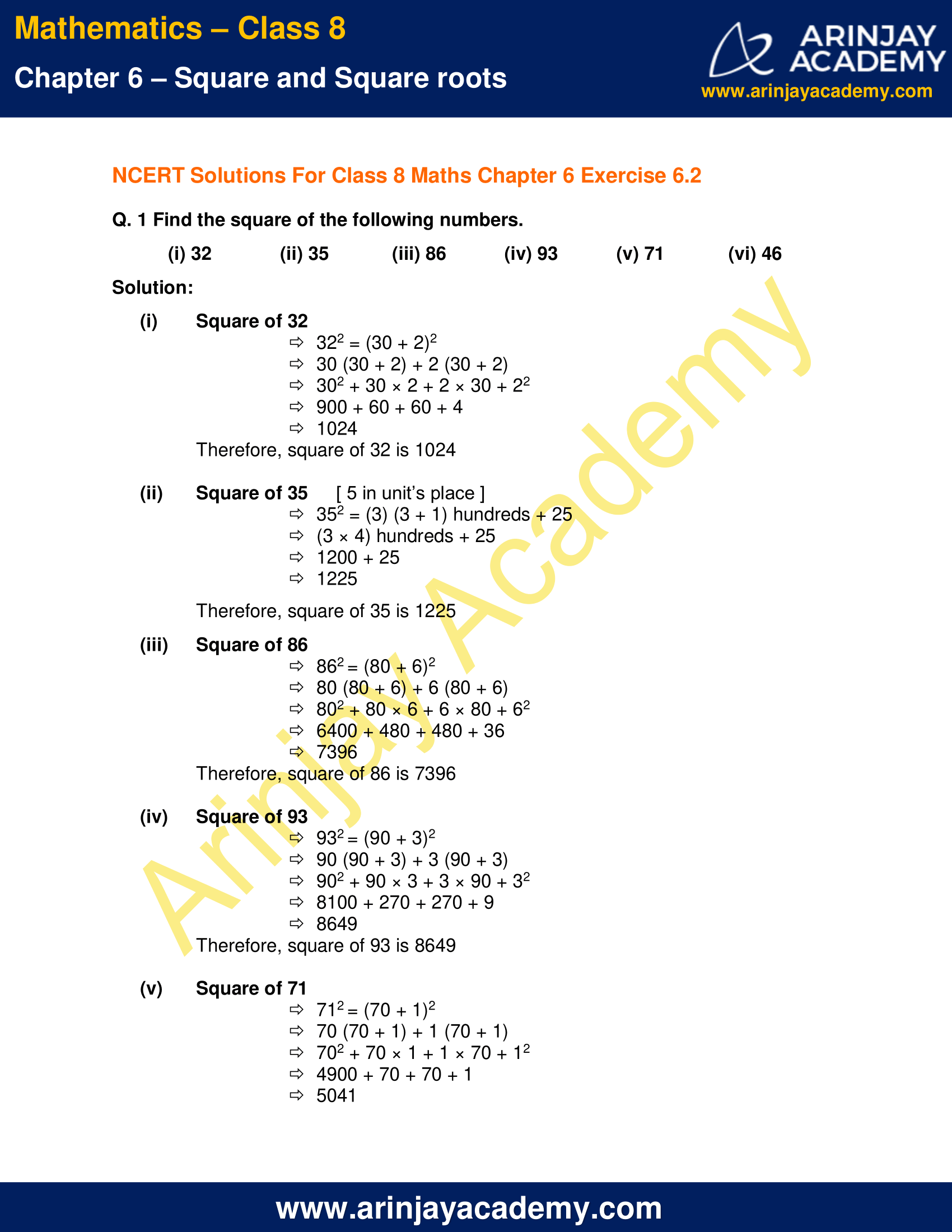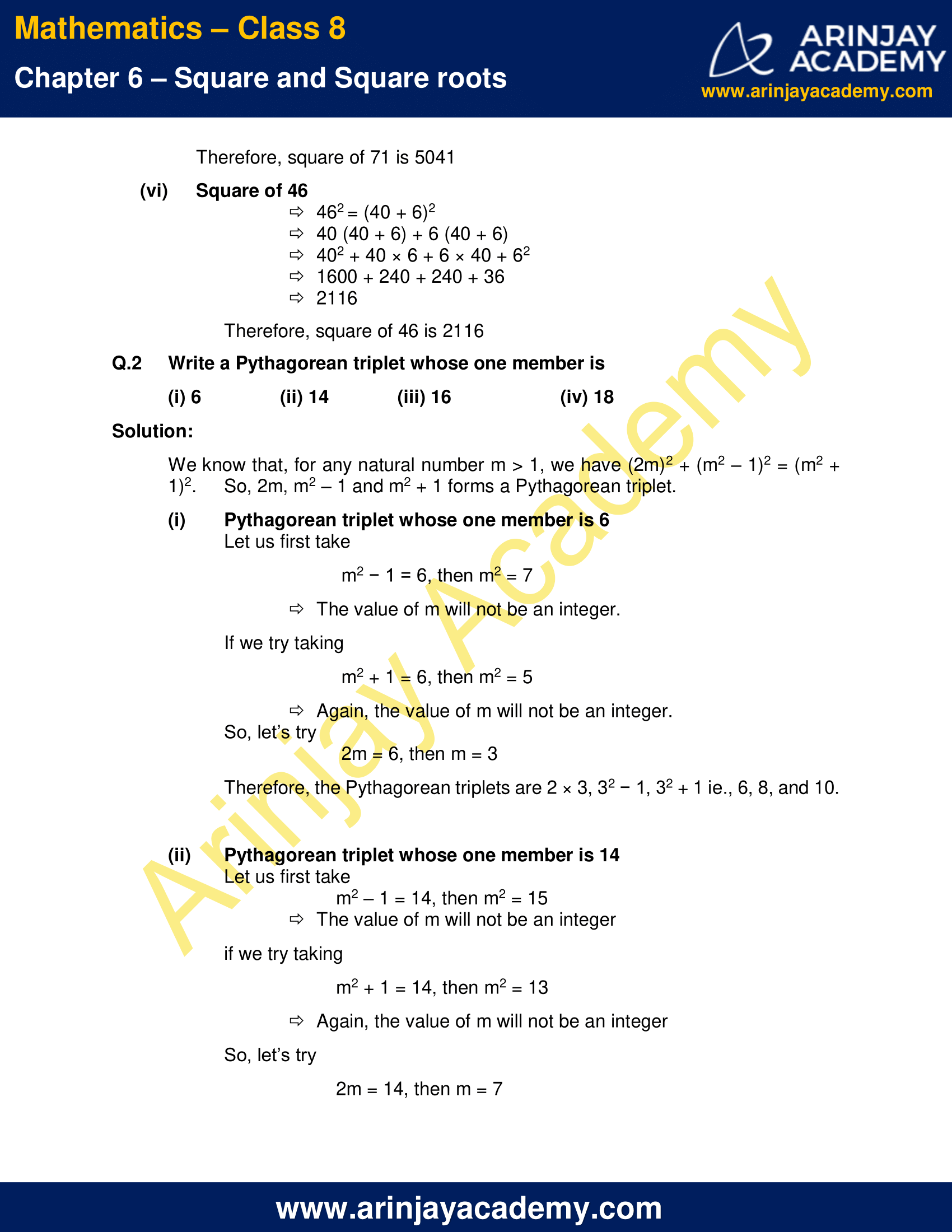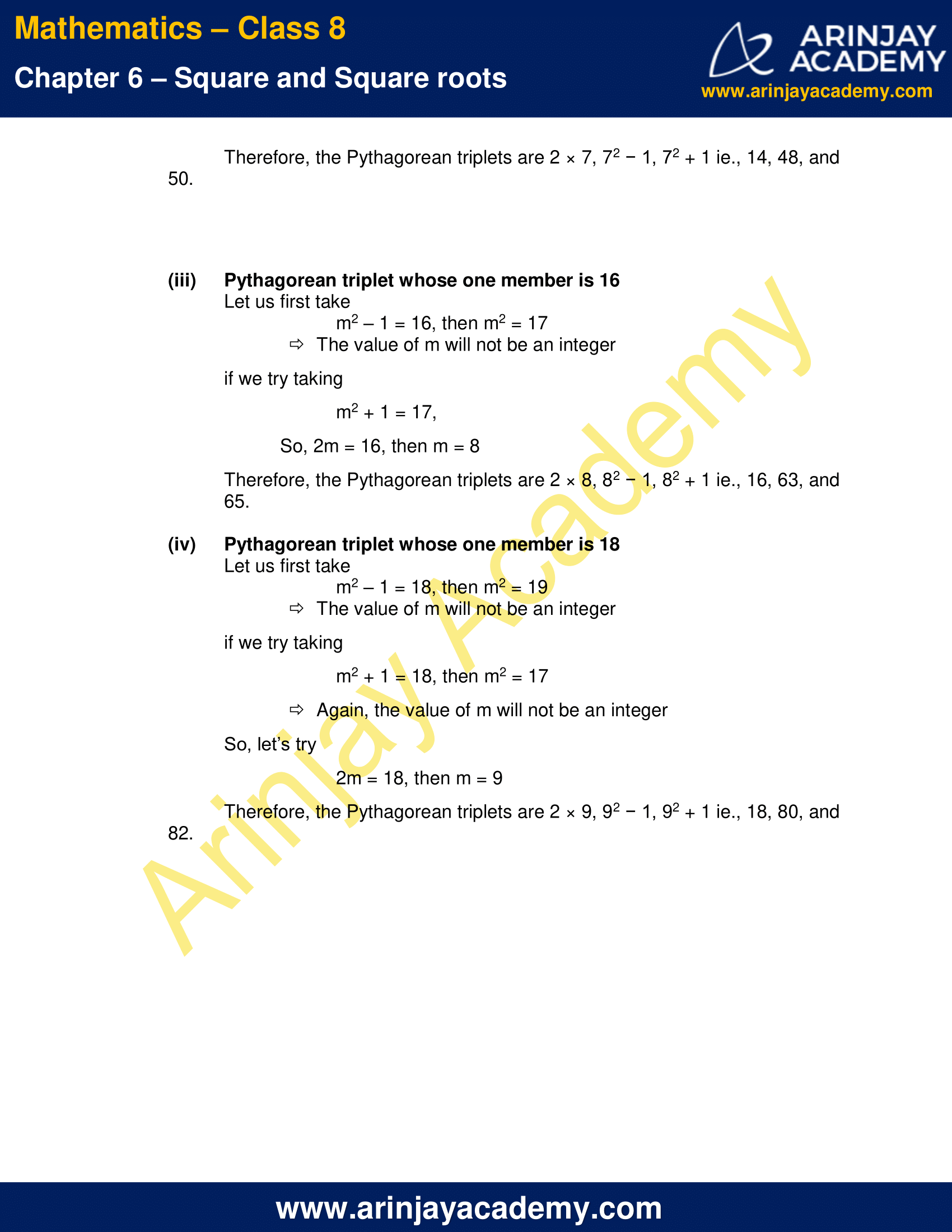# NCERT Solutions for Class 8 Maths Chapter 6 Exercise 6.2

NCERT Solutions for Class 8 Maths Chapter 6 Exercise 6.2 – Square and Square roots, has been designed by the NCERT to test the knowledge of the student on the topic – Finding the Square of a Number

• Other patterns in squares
• Pythagorean triplets

### NCERT Solutions for Class 8 Maths Chapter 6 Exercise 6.2NCERT Solutions for Class 8 Maths Chapter 6 Exercise 6.2

Q. 1 Find the square of the following numbers.

(i) 32
(ii) 35
(iii) 86
(iv) 93
(v) 71
(vi) 46

Solution:

(i) Square of 32

⇒322 = (30 + 2)2
⇒30 (30 + 2) + 2 (30 + 2)
⇒302 + 30 × 2 + 2 × 30 + 22
⇒900 + 60 + 60 + 4
⇒1024

Therefore, square of 32 is 1024

(ii) Square of 35 [ 5 in unit’s place ]

⇒352 = (3) (3 + 1) hundreds + 25
⇒(3 × 4) hundreds + 25
⇒1200 + 25
⇒1225

Therefore, square of 35 is 1225

(iii) Square of 86

⇒862 = (80 + 6)2
⇒80 (80 + 6) + 6 (80 + 6)
⇒802 + 80 × 6 + 6 × 80 + 62
⇒6400 + 480 + 480 + 36
⇒7396

Therefore, square of 86 is 7396

(iv) Square of 93

⇒932 = (90 + 3)2
⇒90 (90 + 3) + 3 (90 + 3)
⇒902 + 90 × 3 + 3 × 90 + 32
⇒8100 + 270 + 270 + 9
⇒8649

Therefore, square of 93 is 8649

(v) Square of 71

⇒712 = (70 + 1)2
⇒70 (70 + 1) + 1 (70 + 1)
⇒702 + 70 × 1 + 1 × 70 + 12
⇒4900 + 70 + 70 + 1
⇒5041

Therefore, square of 71 is 5041

(vi) Square of 46

⇒462 = (40 + 6)2
⇒40 (40 + 6) + 6 (40 + 6)
⇒402 + 40 × 6 + 6 × 40 + 62
⇒1600 + 240 + 240 + 36
⇒2116

Therefore, square of 46 is 2116

Q.2 Write a Pythagorean triplet whose one member is

(i) 6
(ii) 14
(iii) 16
(iv) 18

Solution:

We know that, for any natural number m > 1, we have (2m)2 + (m2 – 1)2 = (m2 + 1)2.
So, 2m, m2 – 1 and m2 + 1 forms a Pythagorean triplet.

(i) Pythagorean triplet whose one member is 6

Let us first take
m2 − 1 = 6, then m2 = 7
⇒The value of m will not be an integer.
If we try taking
m2 + 1 = 6, then m2 = 5
⇒Again, the value of m will not be an integer.
So, let’s try
2m = 6, then m = 3
Therefore, the Pythagorean triplets are 2 × 3, 32 − 1, 32 + 1 ie., 6, 8, and 10.

(ii) Pythagorean triplet whose one member is 14

Let us first take
m2 – 1 = 14, then m2 = 15
⇒The value of m will not be an integer
if we try taking
m2 + 1 = 14, then m2 = 13
⇒Again, the value of m will not be an integer
So, let’s try
2m = 14, then m = 7
Therefore, the Pythagorean triplets are 2 × 7, 72 − 1, 72 + 1 ie., 14, 48, and 50.

(iii) Pythagorean triplet whose one member is 16

Let us first take
m2 – 1 = 16, then m2 = 17
⇒The value of m will not be an integer
if we try taking
⇒m2 + 1 = 17,
So, 2m = 16, then m = 8
Therefore, the Pythagorean triplets are 2 × 8, 82 − 1, 82 + 1 ie., 16, 63, and 65.

(iv) Pythagorean triplet whose one member is 18

Let us first take
m2 – 1 = 18, then m2 = 19
⇒The value of m will not be an integer
if we try taking
m2 + 1 = 18, then m2 = 17
⇒Again, the value of m will not be an integer
So, let’s try
2m = 18, then m = 9
Therefore, the Pythagorean triplets are 2 × 9, 92 − 1, 92 + 1 ie., 18, 80, and 82.

The next Exercise for NCERT Solutions for Class 8 Maths Chapter 6 Exercise 6.3 – Square and Square roots can be accessed by clicking here

Download NCERT Solutions For Class 8 Maths Chapter 6 Exercise 6.2 – Square and Square roots